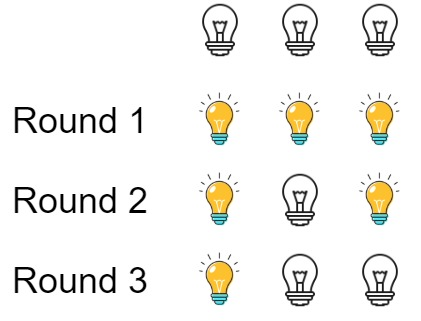## Algorithm

Problem Name: 319. Bulb Switcher

There are `n` bulbs that are initially off. You first turn on all the bulbs, then you turn off every second bulb.

On the third round, you toggle every third bulb (turning on if it's off or turning off if it's on). For the `ith` round, you toggle every `i` bulb. For the `nth` round, you only toggle the last bulb.

Return the number of bulbs that are on after `n` rounds.

Example 1:```Input: n = 3
Output: 1
Explanation: At first, the three bulbs are [off, off, off].
After the first round, the three bulbs are [on, on, on].
After the second round, the three bulbs are [on, off, on].
After the third round, the three bulbs are [on, off, off].
So you should return 1 because there is only one bulb is on.```

Example 2:

```Input: n = 0
Output: 0
```

Example 3:

```Input: n = 1
Output: 1
```

Constraints:

• `0 <= n <= 109`

## Code Examples

### #1 Code Example with Java Programming

```Code - Java Programming```

``````
class Solution {
public int bulbSwitch(int n) {
return (int) Math.sqrt(n);
}
}
``````
Copy The Code &

Input

cmd
n = 3

Output

cmd
1

### #2 Code Example with Javascript Programming

```Code - Javascript Programming```

``````
const bulbSwitch = function(n) {
return Math.floor(Math.sqrt(n));
};
``````
Copy The Code &

Input

cmd
n = 0

### #3 Code Example with Python Programming

```Code - Python Programming```

``````
class Solution:
def bulbSwitch(self, n):
return int(n ** 0.5)
``````
Copy The Code &

Input

cmd
n = 1

Output

cmd
1